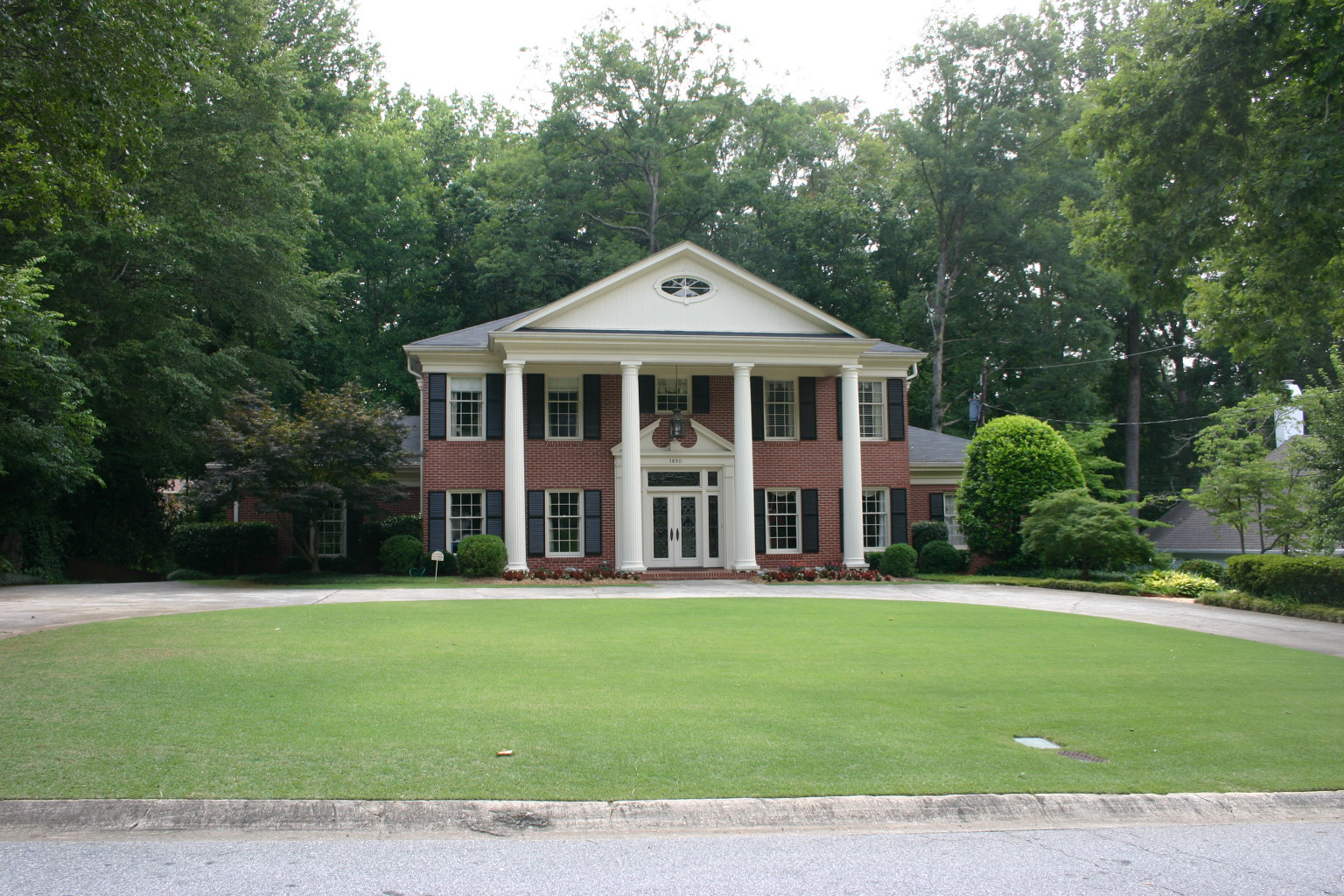# Lawn – Measuring Area (Square Footage)Q: Is there a way to determine the square footage of our lawn? The bottle of weed killer that attaches to the hose said it covers 5000 square feet.

A: When caring for a lawn, its size is a basic fact you need to know.

Application rates for all fertilizers and weedkillers are based on applying a certain amount for each 1000 square feet.

Here’s how to approximately measure the area. You’ll need several empty soft drink cans, a pencil, and a piece of paper:

• Roughly sketch the lawn and divide it into rectangles.

• Put a can on each corner of each rectangle.

• Walk from can to can. Count your paces between each can, and mark the figure on the sketch. Assuming your pace is 2.5 feet long, multiply the number of steps by 2.5 to find the length, in feet, of the sides of each rectangle. If you have a longer or shorter stride, adjust your calculation accordingly.

• For each rectangle, multiply the length of the short side by the length of the long side. This tells you the number of square feet in that rectangle.

• Add the area of all rectangles together to find the total square footage of the lawn.